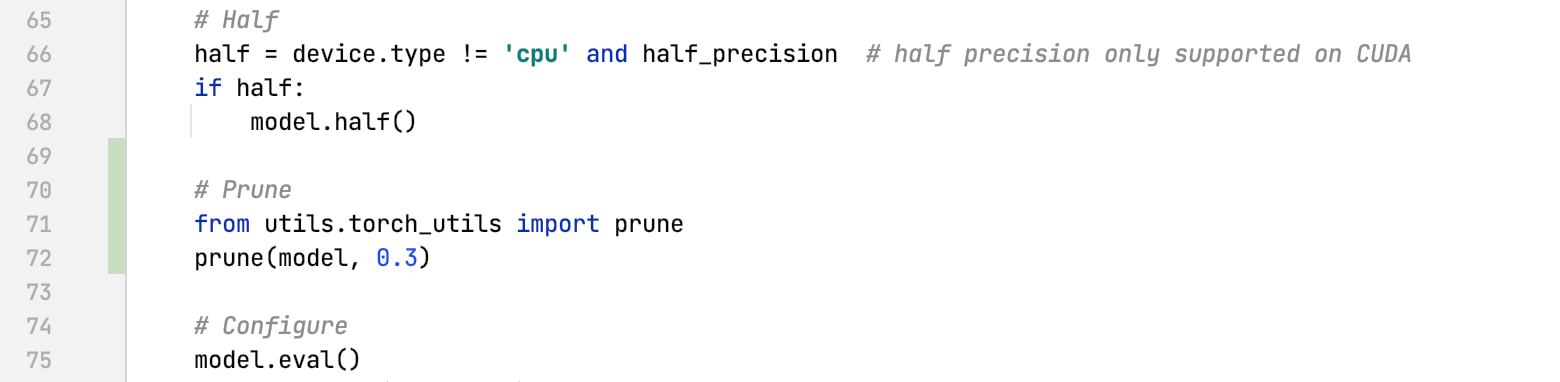Model Pruning/Sparsity

📚 This guide explains how to apply pruning to YOLOv5 🚀 models.

Before You Start

Clone YOLOv5 repo and install requirements.txt dependencies, including Python>=3.8 and PyTorch>=1.7.

\$ git clone https://github.com/ultralytics/yolov5  # clone repo
\$ cd yolov5
\$ pip install -r requirements.txt  # install

Test YOLOv5x on COCO (default)

This command tests YOLOv5x on COCO val2017 at image size 640 pixels to establish a nominal baseline. yolov5x.pt is the largest and most accurate model available. Other options are yolov5s.pt, yolov5m.pt and yolov5l.pt, or you own checkpoint from training a custom dataset ./weights/best.pt. For details on all available models please see our README table.

\$ python test.py --weights yolov5x.pt --data coco.yaml --img 640 --iou 0.65

Default output:

YOLOv5 🚀 v4.0-174-g9c803f2 torch 1.8.0+cu101 CUDA:0 (Tesla V100-SXM2-16GB, 16160.5MB)

Fusing layers...
Model Summary: 476 layers, 87730285 parameters, 0 gradients, 218.8 GFLOPS
val: Scanning '../coco/val2017.cache' images and labels... 4952 found, 48 missing, 0 empty, 0 corrupted: 100% 5000/5000 [00:00<00:00, 50901747.57it/s]

Class      Images      Labels           P           R      mAP@.5  mAP@.5:.95: 100% 157/157 [01:20<00:00,  1.95it/s]
all        5000       36335       0.749       0.619        0.68       0.486
Speed: 5.2/1.6/6.8 ms inference/NMS/total per 640x640 image at batch-size 32  < -------- speed

Evaluating pycocotools mAP... saving runs/test/exp/yolov5x_predictions.json...
Average Precision  (AP) @[ IoU=0.50:0.95 | area=   all | maxDets=100 ] = 0.501  < -------- mAP
Average Precision  (AP) @[ IoU=0.50      | area=   all | maxDets=100 ] = 0.687
Average Precision  (AP) @[ IoU=0.75      | area=   all | maxDets=100 ] = 0.544
Average Precision  (AP) @[ IoU=0.50:0.95 | area= small | maxDets=100 ] = 0.338
Average Precision  (AP) @[ IoU=0.50:0.95 | area=medium | maxDets=100 ] = 0.548
Average Precision  (AP) @[ IoU=0.50:0.95 | area= large | maxDets=100 ] = 0.637
Average Recall     (AR) @[ IoU=0.50:0.95 | area=   all | maxDets=  1 ] = 0.378   < -------- mAR
Average Recall     (AR) @[ IoU=0.50:0.95 | area=   all | maxDets= 10 ] = 0.628
Average Recall     (AR) @[ IoU=0.50:0.95 | area=   all | maxDets=100 ] = 0.680
Average Recall     (AR) @[ IoU=0.50:0.95 | area= small | maxDets=100 ] = 0.520
Average Recall     (AR) @[ IoU=0.50:0.95 | area=medium | maxDets=100 ] = 0.729
Average Recall     (AR) @[ IoU=0.50:0.95 | area= large | maxDets=100 ] = 0.826
Results saved to runs/test/exp

Test YOLOv5x on COCO (0.30 sparsity)

We repeat the above test with a pruned model by using the torch_utils.prune() command. We update test.py to prune YOLOv5x to 0.3 sparsity:30% pruned output:

YOLOv5 🚀 v4.0-174-g9c803f2 torch 1.8.0+cu101 CUDA:0 (Tesla V100-SXM2-16GB, 16160.5MB)

Fusing layers...
Model Summary: 476 layers, 87730285 parameters, 0 gradients, 218.8 GFLOPS
Pruning model...  0.3 global sparsity
val: Scanning '../coco/val2017.cache' images and labels... 4952 found, 48 missing, 0 empty, 0 corrupted: 100% 5000/5000 [00:00<00:00, 50901747.57it/s]

Class      Images      Labels           P           R      mAP@.5  mAP@.5:.95: 100% 157/157 [01:17<00:00,  2.01it/s]
all        5000       36335       0.742       0.595        0.66        0.46
Speed: 5.2/1.5/6.7 ms inference/NMS/total per 640x640 image at batch-size 32  < -------- speed

Evaluating pycocotools mAP... saving runs/test/exp/yolov5x_predictions.json...
Average Precision  (AP) @[ IoU=0.50:0.95 | area=   all | maxDets=100 ] = 0.477  < -------- mAP
Average Precision  (AP) @[ IoU=0.50      | area=   all | maxDets=100 ] = 0.667
Average Precision  (AP) @[ IoU=0.75      | area=   all | maxDets=100 ] = 0.525
Average Precision  (AP) @[ IoU=0.50:0.95 | area= small | maxDets=100 ] = 0.313
Average Precision  (AP) @[ IoU=0.50:0.95 | area=medium | maxDets=100 ] = 0.530
Average Precision  (AP) @[ IoU=0.50:0.95 | area= large | maxDets=100 ] = 0.619
Average Recall     (AR) @[ IoU=0.50:0.95 | area=   all | maxDets=  1 ] = 0.366   < -------- mAR
Average Recall     (AR) @[ IoU=0.50:0.95 | area=   all | maxDets= 10 ] = 0.603
Average Recall     (AR) @[ IoU=0.50:0.95 | area=   all | maxDets=100 ] = 0.654
Average Recall     (AR) @[ IoU=0.50:0.95 | area= small | maxDets=100 ] = 0.475
Average Recall     (AR) @[ IoU=0.50:0.95 | area=medium | maxDets=100 ] = 0.709
Average Recall     (AR) @[ IoU=0.50:0.95 | area= large | maxDets=100 ] = 0.794
Results saved to runs/test/exp

In the results we can observe that we have achieved a sparsity of 30% in our model after pruning, which means that 30% of the model's weight parameters in nn.Conv2d layers are equal to 0. Inference time is essentially unchanged, while the model's AP and AR scores a slightly reduced.

Environments

YOLOv5 may be run in any of the following up-to-date verified environments (with all dependencies including CUDA/CUDNN, Python and PyTorch preinstalled):

StatusIf this badge is green, all YOLOv5 GitHub Actions Continuous Integration (CI) tests are currently passing. CI tests verify correct operation of YOLOv5 training (train.py), testing (test.py), inference (detect.py) and export (export.py) on MacOS, Windows, and Ubuntu every 24 hours and on every commit.